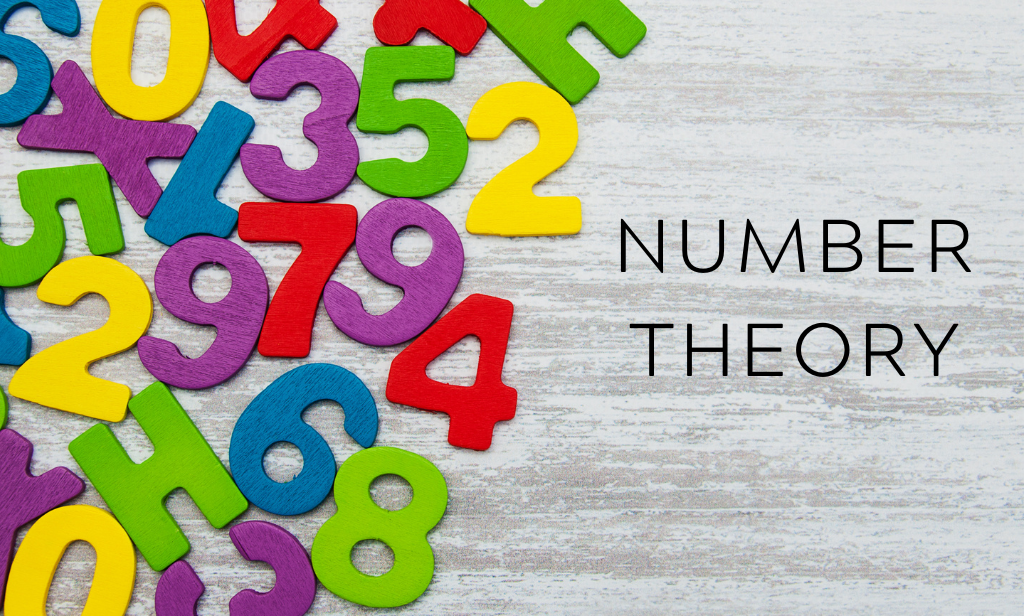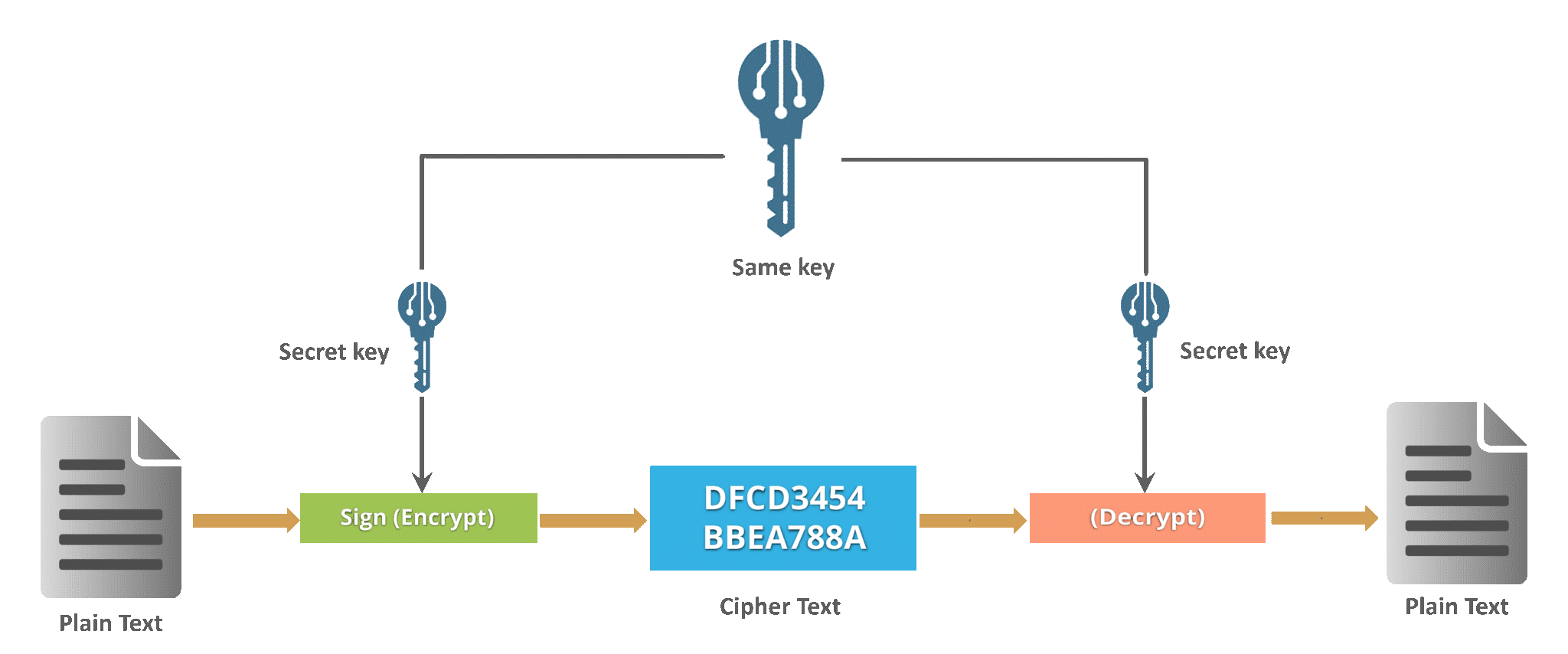Debunking of scopes in Number Theory | Adamas UniversityMathematics

# Debunking of scopes in Number Theory

Introduction:

Number theory has always occupied the significant historical importance from ancient times. It is an integral part of Mathematics not only from ancient times but also in modern times. It has a huge impact in human civilization for its various applicable contributions. It has its origin in ancient India. From ancient times to till the present modern days various mathematicians have given their contributions towards developing this subject for mankind. In this modern arena of scientific development we are in a new beginning after the pandemic caused by the deadly COVID-19. One can say what the helpful impact we can get from Number theory now a days. Rather we can say where and why we can apply Number theory for developing the new era. We have tried to expose some area which have the interest related to Number theory and its applications.

Brief History:

Many great philosophers had contributed various aspects of number theory. Among those was Pythagoras (569 − 500 B.C). His famous Pythagorean theorem led many mathematicians to study squares and sum of squares. He has given us notable Pythagorean triplets. After 200 years, another Greek mathematician Euclid (350 B.C.) drew attention to the prime numbers. Few more studies on natural numbers had been done by Eratosthenes(276B.C.-196B.C.), Nicomachus(C.100) and Diophantus(C.250). Many more mathematicians has contributed in recent times also. But the most significant contributions comes in the field of Cryptography and prime numbers development.

Significant Applications:

There are significant applications of number theory in various fields which has an important impact in real world. Linear Diophantine equation is one of the important topic of number theory which has various applications in modern day life. This equation is of the form  where  ,   are positive integers. This equation has a solution if  divides. If we have n numbers of variables then we can solve the linear Diophantine equation by applying the process for two variables n times. If the Diophantine equation has n unknowns then it is of the form  where   are the variables.  This we can use in balancing chemical equations, determination of molecular formula and also for finding structure of viruses. In chemical equation if we consider an equation  then after balancing by Diophantine equation we can get . Here we can get two integers 2 and 1 for hydrogen and oxygen resulting 2 for water.

Another significant application in the field of number theory is encryption and decryption of messages. Here we use prime number concept and congruence relation together simultaneously.

The significance of coding and decoding increases time to time. In defence, the secret communication plays a key role. Now a days the privacy of data is needed for bank and any other financial transactions. In twenty first century, communications through social media creates a huge impact in our daily life. Essence of secured and encrypted medium is a vital issue for these types of communications. Hence there is a great deal of interest in the techniques of making messages unintelligible to everyone except the intended receiver. Many mathematicians and computer scientists have given their important contribution towards the growth of the discipline devoted to secrecy systems called cryptography. Cryptography is the part of cryptology that deals with design and application of secrecy system and cryptoanalysis is aimed at breaking these systems. Actually the word cryptography is thecombination of two Greek words ‘Krypto’means ‘hidden’and ‘Graphein’means ‘to write’.  In simple words, a message that is to be altered into a secret form is called plaintext while after transformation to a secret form, these messages is said to be ciphertext. The process of converting a plaintext to a ciphertext is called encrypting and the reverse process

of converting from ciphertext to plaintext is said to be decrypting or deciphering. A cipher is a method for altering a plaintext message to ciphertext by changing the letters of plaintext using a transformation. Various concepts of number theory are being applied in the process of encryption and decryption. The most widely used public key cipher known as RSA, is the. In the year 1978, RSA was proposed by Ronald Rivest, Adi Shamir and Leonard Adleman. This public key cipher is based on modular exponentiation.

Impact after COVID-19:

We know that the deadly Covid-19 has affected our life in last two three years very badly. People are getting affected by this virus and the rate of mortality is persisting its significant figure in each wave after wave. Although the biologist, virologist, doctors and scientists are continuously giving their effort for inventing various medicines and vaccines all over the world but we are still very far from eradicating this virus from our society like before. In this process of inventing those vaccines they are using few mathematical calculation by means of number theory.

Again this critical scenario lead us to another difficult part of our society which is social distancing. This social distancing leads us to cashless and online transactions in various places. Now we can see many online payment platform in many shops and digital shopping is fully standing upon this online transactions. This type of transactions are touch free to others and it helps us to maintain social distancing up to some limit. But the main background of this type of transaction is security. The process of encryption and decryption assured us for this type of security. The process of encryption and decryption totally depends on cryptography which is another useful and impactful application of number theory.Here we can transfer plain text to cipher text and vice versa. This aspect of number theory have huge impact in our society.

Although it is quit impossible to illustrate those aspects of number theory very briefly but from this above small discussion reader can understand the usefulness and significance of studying number theory in their course outline. It is also an interdisciplinary area of study which helps a mathematics student and computer science student. Now a days advance number theory and cryptography is in the course curriculum of various academic institutes. But to study those subjects a student should have a clear concept of some basic topics of mathematics like divisibility, greatest common divisor and prime number etc. Those concepts are very much clear for the students from very beginning of their study.

Conclusion:

The most conclusive part of this blog for the reader is to understand the concept of number theory with their uses and to make themselves interested in studying number theory.

Visited 85 times, 1 Visit today

Chemical balancing, Ciphertext, Cryptography, Diophantine equations, Number Theory, Plaintext